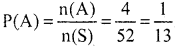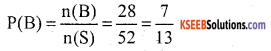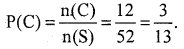# KSEEB Solutions for Class 10 Maths Chapter 11 Introduction to Trigonometry Additional Questions

Students can Download Class 10 Maths Chapter 11 Introduction to Trigonometry Additional Questions, Notes Pdf, KSEEB Solutions for Class 10 Maths helps you to revise the complete Karnataka State Board Syllabus and score more marks in your examinations.

## Karnataka State Syllabus Class 10 Maths Chapter 11 Introduction to Trigonometry Additional Questions

I. Multiple Choice Questions:

Question 1.
How many faces does a coin has
a. 1
b. 2
c. 3
d. 4
b. 2Question 2.
How many faces does a die have
a. 1
b. 2
c. 6
d. 4
c. 6

Question 3.
What is the probability of getting a head on throwing an coin.
a. 0
b. $$\frac{1}{3}$$
c. $$\frac{1}{2}$$
d. 1
c. $$\frac{1}{2}$$

Question 4.
Which of the following cannot be the probability of an «vent.
a. $$\frac{1}{2}$$
b. 10%
c. 0.7
d. $$\frac{3}{2}$$
b. 10%

Question 5.
Two coins are tossed simultaneously the probability of getting a head on only one of the two coins.
a. $$\frac{1}{2}$$
b. $$\frac{2}{3}$$
c. $$\frac{1}{4}$$
d. $$\frac{3}{4}$$
a. $$\frac{1}{2}$$

Question 6.
A die is thrown once the probability of getting an even prime number
a. $$\frac{1}{2}$$
b. $$\frac{1}{3}$$
c. $$\frac{1}{6}$$
d. $$\frac{1}{4}$$
c. $$\frac{1}{6}$$Question 7.
A die is thrown once the probability of getting a number less than 3.
a. $$\frac{1}{2}$$
b. $$\frac{1}{3}$$
c. $$\frac{1}{6}$$
d. $$\frac{5}{6}$$
b. $$\frac{1}{3}$$

Question 8.
Two coins are tossed simultaneously. What is the number of all possible out comes
a.6
b. 2
c. 8
d. 4
d. 4

Question 9.
The probability of getting a perfect square number from the numbers 1 to 10 is.
a. $$\frac{3}{10}$$
b. $$\frac{1}{2}$$
c. $$\frac{2}{5}$$
d. $$\frac{1}{5}$$
a. $$\frac{3}{10}$$

Question 10.
The probability of getting a rotten eggs in a lot of 400 eggs is 0.035 the number of rotten eggs in the lot is
a. 7
b. 14
c. 21
d. 28
b. 14

Question 11.
A die is thrown once the probability of getting a number 3 or 4 is
a. $$\frac{1}{3}$$
b. $$\frac{2}{3}$$
c. 0
d. 1
a. $$\frac{1}{3}$$

Question 12.
In a throw of a pair of dice, the probability of getting a doublet is
a. $$\frac{1}{2}$$
b. $$\frac{1}{3}$$
c. $$\frac{1}{6}$$
d. $$\frac{5}{12}$$
c. $$\frac{1}{6}$$Question 13.
A card is drawn from a well shuffled deck of playing cards. The probability of drawing a red face card is
a. $$\frac{1}{26}$$
b. $$\frac{3}{26}$$
c. $$\frac{4}{26}$$
d. $$\frac{1}{13}$$
b. $$\frac{3}{26}$$

Question 14.
The probability of getting 53 Sundays in a leap year is
a. $$\frac{1}{7}$$
b. $$\frac{2}{7}$$
c. $$\frac{4}{7}$$
d. $$\frac{5}{7}$$
b. $$\frac{2}{7}$$

Question 15.
A letter of english alphabet is chosen at random. The probability that the letter is a consonant is
a. $$\frac{5}{26}$$
b. $$\frac{20}{26}$$
c. $$\frac{21}{26}$$
d. $$\frac{1}{26}$$
c. $$\frac{21}{26}$$

Question 16.
A coin is tossed 1000 times and 560 times a “head” occurs the probability of occurence of a head in this case is
a. 0.5
b. 0.56
c. 0.44
d. 0.056
b. 0.56

Question 17.
A die is thrown once the probability of getting a even number
a. $$\frac{2}{3}$$
b. $$\frac{1}{2}$$
c. $$\frac{2}{6}$$
d. $$\frac{1}{2}$$
d. $$\frac{1}{2}$$

Question 18.
Probability of an a impossible event is
a. 0
b. 1
c. $$\frac{1}{2}$$
d. $$\frac{3}{2}$$
a. 0

Question 1.
Write the probability of sure event or. certain event
1 (one)Question 2.
Write the probability of an impossible event.
zero (0)

Question 3.
Write the sum of the probabilities of all the elementary events of an experiment.
one (1)

Question 4.
Define elementary event.
An event having only one out comes of the experiment.

Question 5.
Probability of an event lies between _______
0 and 1

Question 6.
Write the formula for calculating the probability of occurence of an event.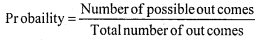Question 7.
From a well shuffled pack of cards, a card is drawn at random. Find the probabilities of getting a black queen.
n(S) = 52, number of black queens = 2
∴ n(A) = 2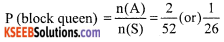Question 8.
If PE. = 0.05, what is the probability of’notE’
P(E) = 0.05, P($$\overline{\mathbf{E}}$$) = ?
P(E) + P(not E) = 1
P(not E) = 1 – P(E) = 1 – 0.05
= 0.95Question 9.
What is the probability of getting no head when two coins are tossed simultaneously?
n(S) = {HH, TT, HT, TH} n(S) = 4 and n(A) = 1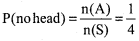Question 10.
Someone is asked to choose a number from 1 to 100. What is the probability of it being a prime number.
n(S) = 100, total prime number between 0 and 100 = 25 n(A) = 25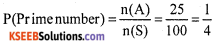Question 11.
A card is drawn at random from a well shuffled pack of 52 playing cards. Find the probability of getting neither a red card nor a queen.
n(S) = 52, Number of red cards and queen = 28
Number of favourable out comes = 52 – 28 = 24
P (getting neither red card nor a queen)
= $$\frac{24}{56}=\frac{6}{13}$$

Question 12.
20 tickets on Which numbers 1 to 20 are written, are mixed throughly and then a ticket is drawn at random out of them. Find the probability that number on the drawn ticket is a multiple of 3 or 7.
n(S) = 20 multiple of 3 or 7 = {3, 6, 9, 12, 15, 18, 7, 14} n(A) = 8
P (ticket multiple 3 or 7)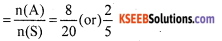Question 1.
A bag contains 6 red balls and some blue balls. If the probability of drawing a blue ball from the bag is twice that of a red ball. Find the number of blue balls in the bag.
Number of red balls = 6 and Let the number blue balls = x
Total number of balls = x + 6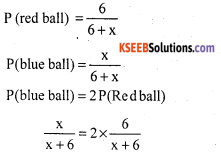x = 12
Hence, number of blue balls is 12.Question 2.
There are 30 boys and 25 girls in a class. A student is chosen at random. Find the probability that the chosen student is a
i. boy
ii. girl
Total number of students = 30 + 25 = 55
n(S) = 55
(i) Let A be the event of choosing a boy, n(A) = 30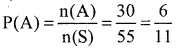(ii) Let B be the event of choosing a girl n(B) = 25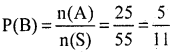Question 3.
A random experiment has only three pairs of mutually exclusive events among A, B and C. If PA. = $$\frac{3}{2}$$ P(B) and P(C) = $$\frac{1}{2}$$ P(A), Find P(B).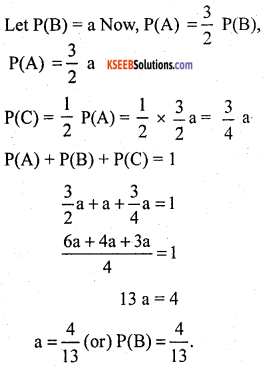Question 4.
If A is an event of a random experiment such that P(A) : P($$\bar{A}$$) = 5 : 11, then find (i) P(A) and P($$\bar{A}$$) = ?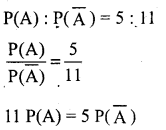11 P(A) = 5 {1 – P(A)}
11 P(A) = 5 – 5 P(A)
11 P(A) + 5 P(A) = 5
16 P(A) = 5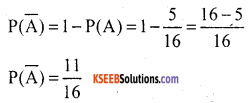Question 5.
Nine rotten mangoes are mixed with 30 good ones. One mango is chosen at random. What is the probability of choosing a
i. good mango
ii. rotten mango.
Total number of mangoes = n(S)
= 30 + 9. ∴ n(S) = 39

(i) Let ‘A’ be the event of good mangoes.
n(A) = 30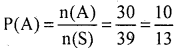(ii) Let ‘B’ be the event of rotten mangoes. n(B) = 9.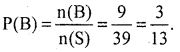Question 6.
The Probability of picking non-defective item for sample is $$\frac{7}{12}$$. Find the Probability of picking a defective item.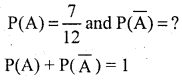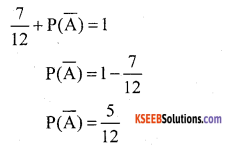Question 7.
There are 2 red and 2 yellow flowers in a basket. A child picks up at random three flowers, what is the probability of picking up both the yellow flowers.
Total number of flowers = 2 Red + 2 Yellow = 4
3 flowers from out 4 flowers drawn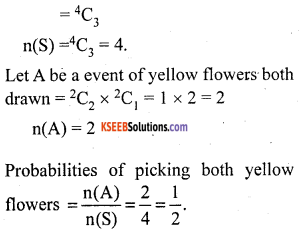Question 8.
Three cards are drawn at random from a pack of 52 cards. What is the probability that all the three cards are kings.
There are 52 cards, three cards are drawn,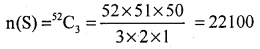Let A be the event when all 3 cards chosen are kings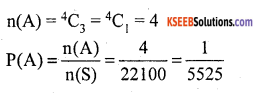Question 9.
One card is drawn from a pack of 52 cards, each of the 52 cards being equally likely to be drawn. Find the probability that the card drawn is
i. an ace
ii. either red or king
iii. a face card.